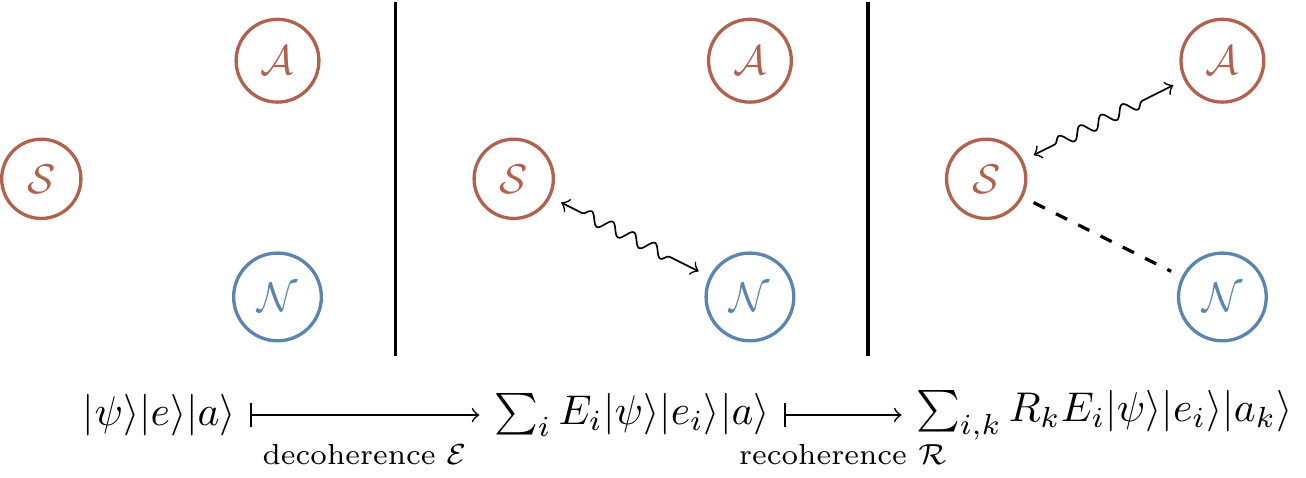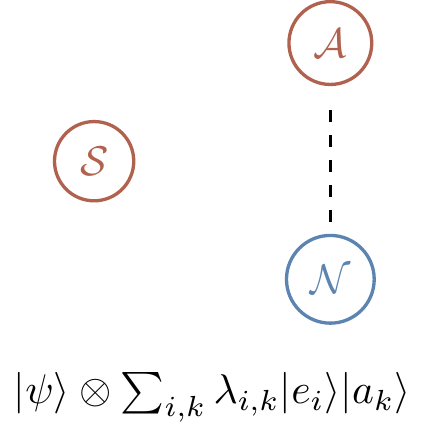## 13.4 Quantum errors

The most general qubit-environment interaction is of the form \begin{aligned} |0\rangle|e\rangle &\longmapsto |0\rangle|e_{00}\rangle + |1\rangle|e_{01}\rangle \\|1\rangle|e\rangle &\longmapsto |1\rangle|e_{10}\rangle + |0\rangle|e_{11}\rangle \end{aligned} where the states of the environment are neither normalised nor orthogonal. This leads to decoherence \begin{aligned} \Big( \alpha|0\rangle + \beta|1\rangle \Big) |e\rangle \longmapsto & \Big( \alpha|0\rangle + \beta|1\rangle \Big) \frac{|e_{00}\rangle+|e_{11}\rangle}{2} \\+& \Big( \alpha|0\rangle - \beta|1\rangle \Big) \frac{|e_{00}\rangle-|e_{11}\rangle}{2} \\+& \Big( \alpha|1\rangle + \beta|0\rangle \Big) \frac{|e_{01}\rangle+|e_{10}\rangle}{2} \\+& \Big( \alpha|1\rangle - \beta|0\rangle \Big) \frac{|e_{01}\rangle-|e_{10}\rangle}{2}. \end{aligned} which can be written as |\psi\rangle|e\rangle \longmapsto \mathbf{1}|\psi\rangle|e_{\mathbf{1}}\rangle + Z|\psi\rangle |e_Z\rangle +X|\psi\rangle |e_X\rangle + Y|\psi\rangle |e_Y\rangle.

The intuition behind this expression is that four things can happen to the qubit:

1. nothing (\mathbf{1})
2. phase-flip (Z)
3. bit-flip (X)
4. both bit-flip and phase-flip (Y).

This is certainly the case when the states |e_{\mathbf{1}}\rangle, |e_X\rangle, |e_Y\rangle and |e_Z\rangle are mutually orthogonal, but if this is not so then we cannot perfectly distinguish between the four alternatives.261

The important thing is the discretisation of errors, and the fact that we can reduce quantum errors in this scenario to two types: bit-flip errors X, and phase-flip errors Z.

In general, given n qubits in state |\psi\rangle, and an environment in state |e\rangle, the joint evolution can be expanded as262 |\psi\rangle|e\rangle \longmapsto \sum_{i=1}^{4^n} E_i|\psi\rangle|e_i\rangle, where the E_i are the n-fold tensor products of the Pauli operators and the |e_i\rangle are the corresponding states of the environment (which, again, are not assumed to be normalised or mutually orthogonal). For example, in the case n=5, a typical operator E_i may look like X\otimes Z \otimes \mathbf{1}\otimes \mathbf{1}\otimes Y \equiv XZ\mathbf{1}\mathbf{1}Y. We say that such an E_i represents an error consisting of the bit error (or X error) on the first qubit, phase error (or Z error) on the second qubit, and both bit and phase error (or Y error) on the fifth qubit.263

At first glance, once our bunch of qubits, initially in state |\psi\rangle, gets entangled with the environment |\psi\rangle|e\rangle \longmapsto \sum_i E_i|\psi\rangle|e_i\rangle the situation looks rather hopeless — we do not have any control over the environment. However, there is a way around this. We can couple the qubits to an auxiliary system that we do control (an ancilla), and then attempt to transfer the qubits–environment entanglement to a qubits–ancilla entanglement. In other words, we prepare the ancilla in some prescribed state |a\rangle and try to undo the decoherence \mathcal{E} using some recovery or recoherence operator \mathcal{R} that acts as |\psi\rangle|a\rangle \longmapsto \sum_k R_k|\psi\rangle|a_k\rangle. Thus decoherence followed by recoherence acts as \begin{aligned} \mathcal{R}\mathcal{E}\colon |\psi\rangle|e\rangle|a\rangle &\longmapsto \sum_i E_i|\psi\rangle|e_i\rangle|a\rangle \\&\longmapsto \sum_{i,k} R_kE_i |\psi\rangle|e_i\rangle|a_k\rangle \end{aligned} which we can also express in a diagram, as in Figure 13.3.Figure 13.3: The initial state undergoing decoherence followed by recoherence. Here \mathcal{S} is the system of qubits that we want to work with, \mathcal{N} is the environment, and \mathcal{A} is the ancilla that we introduce. The squiggly arrow represents interactions, and the dashed line represents entanglement.

But for this to help us, we need to end up with in a state where the ancilla and the environment are entangled with one another, and the qubit is entangled with nothing, i.e. a state of the form |\psi\rangle\otimes(\text{some entangled state of the ancilla and environment}) as shown in Figure 13.4Figure 13.4: The desired outcome of the decoherence–recoherence process.

Ideally we would like this to hold for all states |\psi\rangle, but this turns out to be too much to ask: as we shall see in a moment, we will have to confine our recoverable states to those that belong to a subspace called the codespace. But then at least for these states we expect to have \sum_{i,k} R_kE_i |\psi\rangle|e_i\rangle|a_k\rangle = \sum_{i,k} |\psi\rangle\otimes\lambda_{ik}|e_i\rangle|a_k\rangle. The ability of R_k to perform the correction like this means that R_kE_i = \lambda_{ik}\mathbf{1} when acting on the codespace states |\psi\rangle. In turn, this means that \begin{aligned} \sum_k (R_k E_j)^\dagger(R_k E_i) &= E_j^\dagger \left(\sum_k R_k^\dagger R_k\right) E_i \\&= E_j^\dagger E_i \\&= \lambda_{jk}^\star\lambda_{ik}\mathbf{1} \end{aligned} which reminds us of the hopefully now-familiar condition for being able to correct a randomly chosen isometry.

Last but not least, note that the recoherence operator \mathcal{R} not only allows us to recover from the errors E_i, but also from any errors that are in the linear span of these. Thus if the errors E_i form a basis in the matrix space — as is the case for the Pauli matrices together with the identity — then once we design an error recovery scheme for the E_i, we will be able to correct any error.

If a quantum error correction method corrects errors E_1 and E_2, then it also corrects any linear combination of E_1 and E_2.

1. We will soon stop warning that this intuition is not entirely accurate, so keep it in mind!↩︎

2. The sum is from i=1 to 4^n because there are 4^n different (tensor products of) Pauli operators acting on n qubits.↩︎

3. One final time: this is not entirely accurate if the corresponding states of the environment are not mutually orthogonal, but it gives the right kind of intuition nonetheless.↩︎